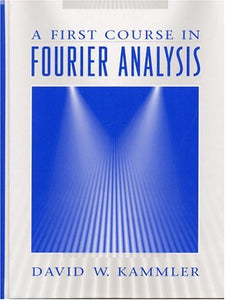# First Course In Fourier Analysis, A

• \$41.35
• Save \$24.16

Attention: For textbook, access codes and supplements are not guaranteed with used items.

This unique book provides a meaningful resource for applied mathematics through Fourier analysis. It develops a unified theory of discrete and continuous (univariate) Fourier analysis, the fast Fourier transform, and a powerful elementary theory of generalized functions and shows how these mathematical ideas can be used to study sampling theory, PDE's, probability, diffraction, musical tones, and wavelets. Providing unified development of (univariate) Fourier analysis for functions on R, T, Z, and P, the book also includes an unusually complete presentation of the Fourier transform calculus. It uses concepts from calculus to present an elementary theory of generalized functions. It also uses the FT calculus and generalized functions to study the (univariate) wave equation, diffusion equation, and diffraction equation. In addition, fine points of the theory are developed. The book also demonstrates real-world applications of Fourier analysis in the chapter on musical tones. A valuable reference on Fourier analysis for a variety of scientific professionals, including Mathematicians, Physicists, Chemists, Geologists, Electrical Engineers, Mechanical Engineers, and others.